Garrett Mayock's Blog

# Non-naive Models for Predicting Stationary Time Series Data

### Following along Managing Supply Chain Operations Chapter 2 using Python, cont'd.Garrett Mayock posted 2019-02-27 22:34:43 UTC

I am reading Managing Supply Chain Operations. Chapter 2 details Forecasting and Demand Management methods. I figured I'd kill two birds with one stone (learning the material and learning Python) by recreating the chapter material in Python. I anticipate answering the chapter exercises in Python as well, but will save that for another blog post.

(Writer's note - the book groups naive and non-naive models for predicting stationary time series data. Naive models are covered in the previous blog post.)

Stationary Series Forecasting

The first time series prediction methods discussed in this book are used for predicting stationary (horizontal) series - series where no trend or seasonal component is apparent in the data. The values fluctuate around a constant mean, even if not all values are exactly the same.

Three types of models are discussed for stationary data: naive models, simple moving average models, and exponential smoothing models. Naive models are covered in the previous blog post.

Moving average model

The moving average model, also denoted MA(N), is a forecast model that smooths out the random fluctuations. N refers to the number of most-recent values in the time series that are accounted for - MA(3) looks at the last 3, MA(10) at the last 10, and so on. Moving average models also provide stable forecasts in the case where there is a shift in the mean - for example, if the advertising promotion I mentioned towards the end of the last blog post was temporary, and led to a permanent increase, the moving average model would be able to reflect this shift over time.

The input demand data is the same as I used in the previous blog post, so we'll use the same code to ingest it:

```import pandas as pd
import matplotlib.pyplot as plt
import numpy as np

df.reset_index(drop=True, inplace=True)
```

Note I'm also importing matplotlib.pyplot and numpy for use later on.

The first task is to calculate the MA(4). Since we are calculating one-step-ahead forecasts, we have to shift the moving average over one - that is, the moving average for week 5 only includes weeks 1-4. We'll use the pandas rolling() and mean() functions to calculate the moving average.

```df['MA(4)'] = np.nan
df['MA(4)'][1:] = df['Demand'].rolling(window=4).mean()[:-1]
```

Note how I use numpy to fill a new column will NaNs before entering in the shifted rolling average. This is because pandas doesn't support filling in parts of columns which don't exist (it will throw a KeyError), and doesn't default to creating a new column in order to do so. This is a feature, not a bug.

Now let's add on the error calculation:

```df['MA(4)_err'] = df['Demand']-df['MA(4)']
```

And take a look at the resulting dataframe:

```print(df)
```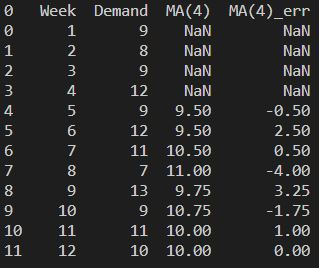We can also print the Mean Absolute Deviation (MAD) as an accuracy measure:

```print("\n Mean Absolute Deviation: \n",abs(df['MA(4)_err']).mean())
```

Returns: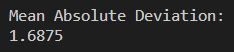Not surprisingly, since the data truly is stationary, the arithmetic mean model from the last blog post still posts the best score of the models we've tried, at a MAD of 1.55. Let's see what this line looks like graphed. Here's the code:

```plt.ylabel('Widget Demand (000s)');
plt.ylim(0,15);
plt.axes().yaxis.grid(linestyle=':');
plt.xlabel('Week');
plt.xlim(0,15);
plt.title('Widget Demand Data');
plt.plot(df['Week'], df['Demand'], marker='o');
plt.plot(df['Week'], df['MA(4)'], marker='D');
plt.legend();
plt.show();
```

And the result: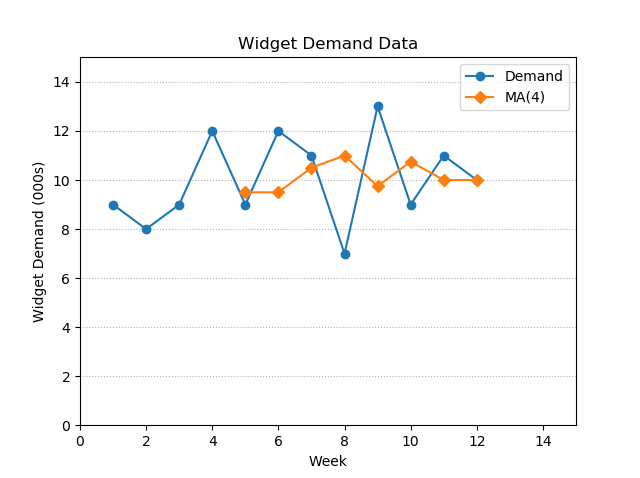Note how I've included a legend this time.

At this point the book notes how to extrapolate the MA(N) forecast more than one step ahead in the future, one simply uses the most recent moving average. So, the forecast for week 13 (created with weeks 9-12 data) will be used for the week 14 forecast if one is still projecting out from week 12 - and it will also be used for week 15, and 16, and so on, as long as one projects from week 12. In other words, it flattens out. I'll make a visualization. First we calculate the average of weeks 9-12:

```m = sum(df['Demand'][-4:])/4
print(m)
```

m = 10.75. Now I'll create a dataframe with the same column header as my previous

```df_multi_step = pd.DataFrame([[m, i+13] for i in range(0,9)], columns=['MA(4)', 'Week'])
print(df_multi_step)
```

You'll see I used list comprehension to populate a dataframe with one column with nine values all equal to m, the "moving" average we calculated for weeks 9-12 above, as well as weeks 13-21. This results in a new dataframe: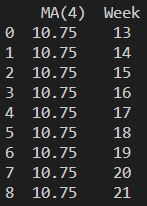We then merge on both columns:

```new_df = pd.merge(df, df_multi_step, on=['MA(4)', 'Week'], how='outer')
print(new_df)
```

Which results in: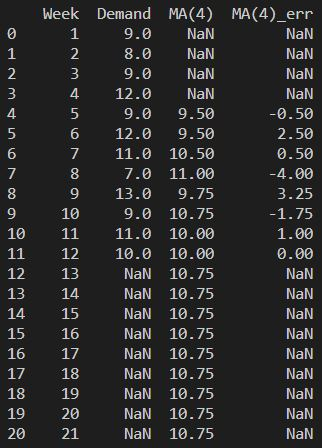Now we can plot it. Since I created a new dataframe, we will have to edit the code from df to new_df. We also have to extend the x axis limit (I choose 25 for example's sake). This is the result: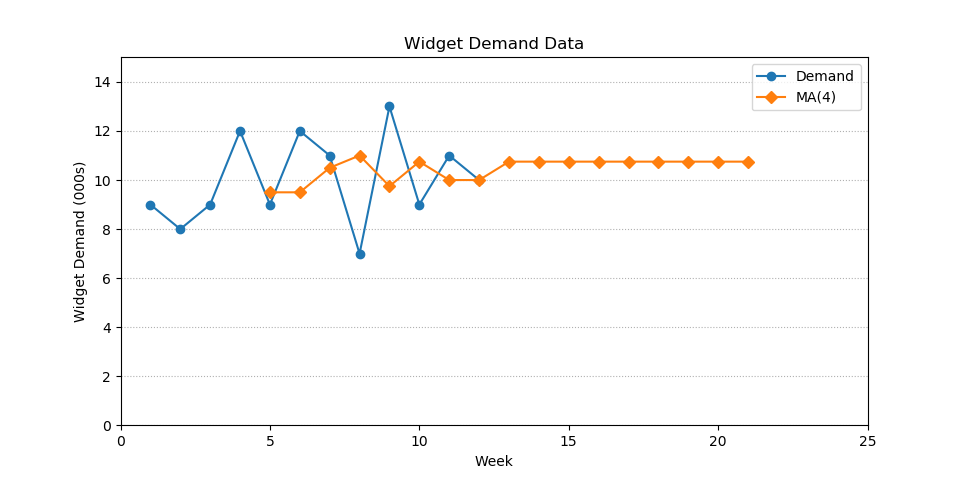The book then begins to discuss the various ways to choose N. Essentially, a smaller N can be subject to recency bias, while a larger N could include irrelevant past information. N should be chosen based on the specific circumstances the user desires.

The book says for actual data, they use a trial-and-error procedure to determine what value of N will be optimal - I will be on the lookout for a better method as I go through the book.

Exponential smoothing model

Next we move on to the exponential smoothing model. This is a model which uses a series of decreasing weights to weigh more-recent weekly demand more heavily than previous weekly demand. In the case of an exponential smoothing model, the progression is geometric, but I suppose other smoothing models could be created with arithmetic progression or harmonic progression - they just wouldn't be exponential smoothing models.

Exponential smoothing models are a tad more complex than the models we've previously discussed. The forecast for a new period has three parts:

• A smoothing constant, 0 < α ≤ 1
• The actual demand of the time series in the previous period
• The forecast demand from the previous period

The equation is: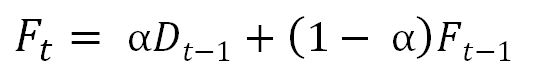We can expand the equation by replacing the forecasts for previous time periods with the value given by the equation, resulting in an expansion that follows this pattern: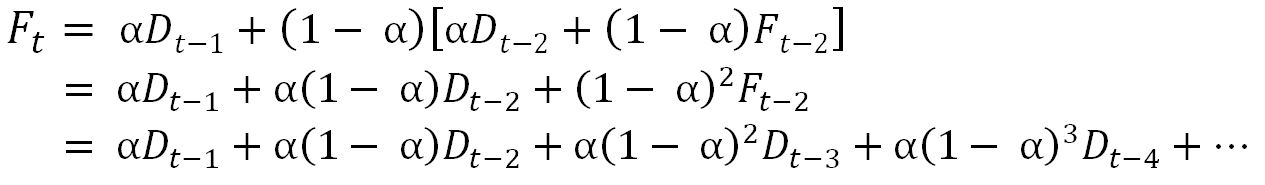However, if we recall the previous blog post, the error is the difference between the forecast and actual demand for a given time period. We can further rearrange the equation above using this information to make a computationally less expensive formula: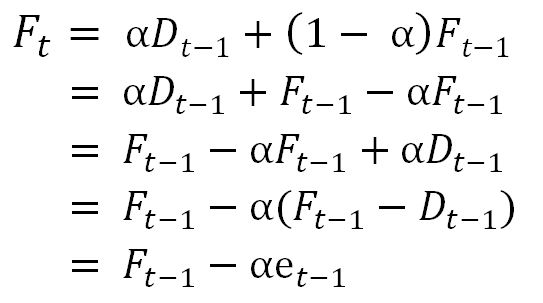Note that e represents the error.

It's important to note that the exponential smoothing model requires an initial forecast assumption - if we extrapolate the above formulas to the forecast for the first period, we'll see it's dependent upon the previous period's demand and forecast. However, those do not exist, which means we can't calculate a forecast for the first period, which means we can't calculate a forecast for the second period, and so on. Therefore, it's common to set the forecast for the first period to the same as demand for the first period, or as the average of a few early periods.

Now I want to calculate this, setting the forecast for the first period equal to demand for the first period, and using an α value of 0.1. First, I'll declare α as "alph", then populate a new column for forecasted values with the demand values for the same week, and then I'll replace all forecasted values after period 1 with the new forecasted values from the exponential smoothing function. I'll also calculate and print the MAD:

```alph = 0.1
df['expo_pred'] = df['Demand'].astype('float')
for i in range(1, len(df['expo_pred'])):
df['expo_pred'][i] = round(alph*df['Demand'][i-1]+(1-alph)*df['expo_pred'][i-1],2)
df['expo_abs_err'] = abs(df['expo_pred']-df['Demand'])
print("\n Mean Absolute Deviation: \n", round(df['expo_abs_err'].mean(),2))
```

Note how I do not include the first week in the calculation of the error. This is because the first week forecast is set to the same as demand, and hence the error is zero, so including it makes the MAD look artificially better than it is.

Which comes out to: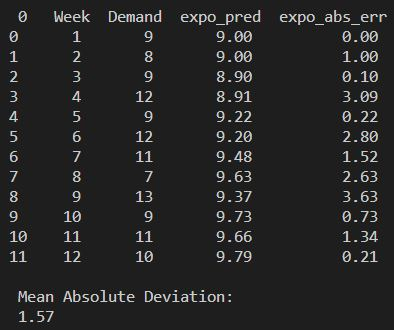Now we'll plot it so show visually how it's nice and smooth. The book only plots the forecasted values from Week 2 on, hence I use the [1:] in the line plot code for the forecast line:

```plt.plot(df['Week'], df['Demand'], marker='o');
plt.plot(df['Week'][1:], df['expo_pred'][1:], marker='D');
```

Note the rest of the code remains the same as the first plot code, to retain formatting.

The result is this: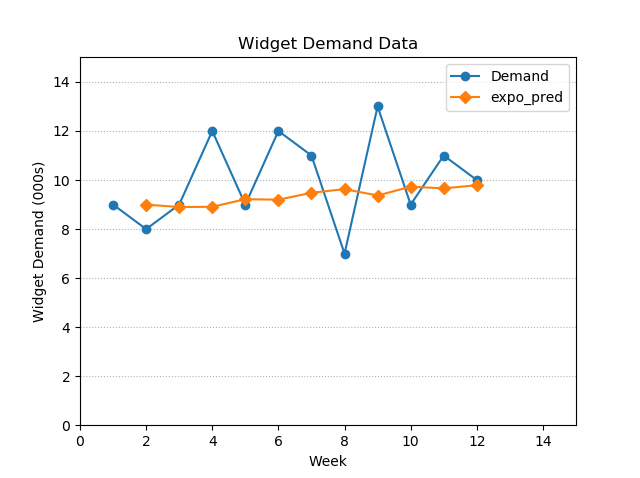Multiple-step-ahead exponentially smooth forecasting follows the same method as the multiple-step-ahead moving average model.

As the last part of the stationary time series data forecasting section of the book, there is a discussion on choosing the optimal α value. Similar to choosing N, a larger α results in more recency bias (a greater weight given to more recent data), whereas a smaller α reacts to paradigm shifts slower but results in smoother forecasts from period to period.

The writers mention that α can be chosen via trial and error. A rule of thumb is that for production applications, α generally rests on or between 0.1 and (0.2 or 0.3).

Using Excel Solver to find an optimal α

Then, they mention we can find an optimal α by using Microsoft Excel Solver (click here for the add-on's activation instructions). For some reason, they use different input data, so I've added that on the 2.3.4_seeking_alpha tab. They also made the first forecast 1 larger than the first demand. The input data is in rows 1 through 18 in the image and Excel file - I've also copied it to rows 20 through 37 in order to be able to run the Solver and show the results simultaneously: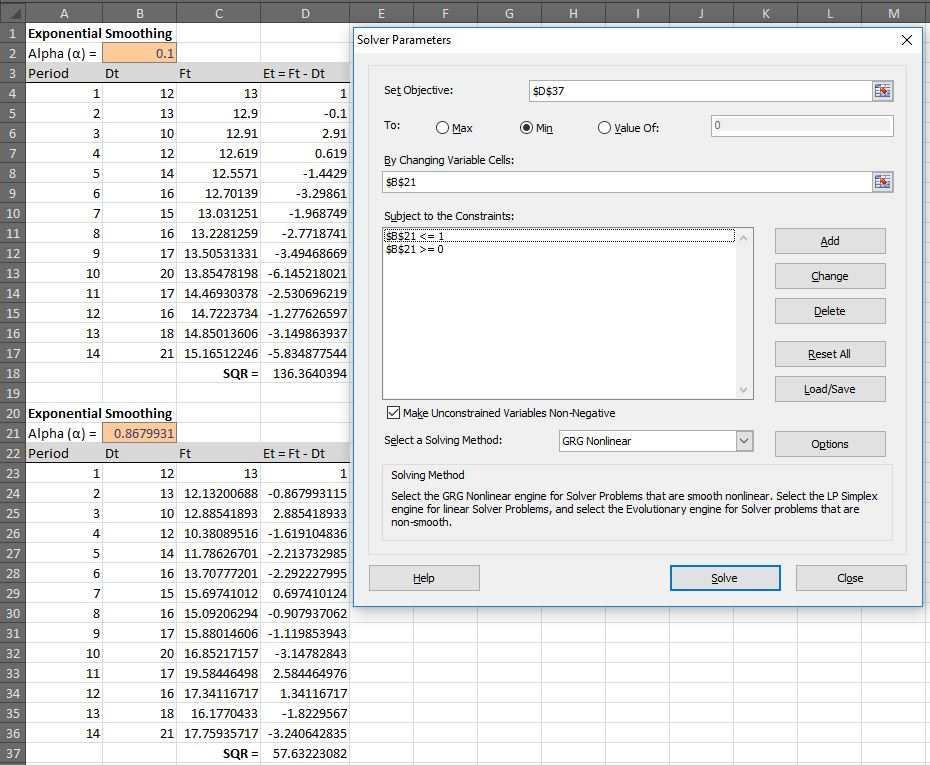Note the constraints.

Replicating the Solver exercise in Python

Now I want to replicate this Solver exercise in Python. I can't just ingest the tab I've made for Solver purposes with similar code as I do the other tab - that's because the format is not easily machine-readable. If I do, it would show all of the information in the range A1:D:37, including the nulls, the second table, and so on. Therefore, I'll design code that knows the table headers are in the third row (index  in Python) and that only ingests the first 14 rows after the headers (because we know we only have 14 weeks of data).

```f2 = pd.read_excel('D:/Keep/Learning/Supply Chain/Managing Supply Chain Operations/xL/chapter_2_instruction.xlsx', sheet_name='2.3.4_seeking_alpha', header=2, index_col=None, nrows=14)
print(df2)
```

And here's how it looks: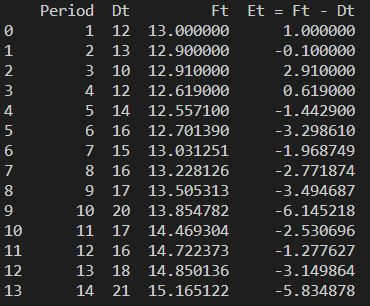So this is a new problem. First I'll define the two formulas in Python to ensure I can recreate the values in the Excel columns. The formulas look like this:

```def exponentialForecast(period, alpha):
if period == 1:
f = 13
else:
f = alpha*df2['Dt'][period-2]+(1-alpha)*exponentialForecast(period-1, alpha)
return f

def exponentialError(period, alpha):
err = exponentialForecast(period, alpha)-df2['Dt'][period-1]
return err
```

I checked these formulas by printing the results (with alpha set to one):

```print("\n Formulaic forecasts:")
for i in range(1,len(df2['Period'])+1):
print(exponentialForecast(i, alph))

print("\n The formula's errors:")
for i in range(1,len(df2['Period'])+1):
print(exponentialError(i, alph))
```

Note how I had to add one to the len for the loop range. That's because range is not inclusive of the final value - a range of (1,14) ends at 13.

They match. Now let's set a variable to equal the total square error:

```sq_err = sum([pow(exponentialError(i, alph),2) for i in range(1,len(df2['Period'])+1)])
print(sq_err)
```

This formula squares the result of the error formula and sums it for all periods. The result is 136.364..., which matches.

Okay, now I just have to minimize sq_err by changing alph within the constraints (0 < α ≤ 1). First, I'll use a loop to calculate values and graph them, so I can visualize it. This code creates a dataframe with the values:

```df3 = []
for i in range (0, 1000):
val = [i/1000, sum([pow(exponentialError(q, i/1000),2) for q in range(1,len(df2['Period'])+1)])]
df3.append(val)
df3 = pd.DataFrame(df3, columns=['alpha_value','squared_error'])
```

And this code plots it:

```plt.ylabel('Squared Error');
plt.ylim(0,max(df3['squared_error']))
plt.axes().yaxis.grid(linestyle=':');
plt.xlabel('Alpha Value');
plt.title('Squared Error Curve by Alpha');
plt.plot(df3['alpha_value'], df3['squared_error']);
plt.legend();
plt.show();
```

The chart looks like this: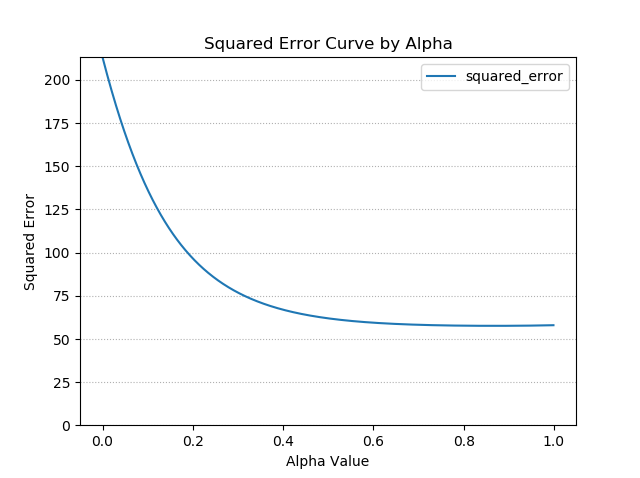I'll change the ylim to focus on the area around the minimum, (57,59):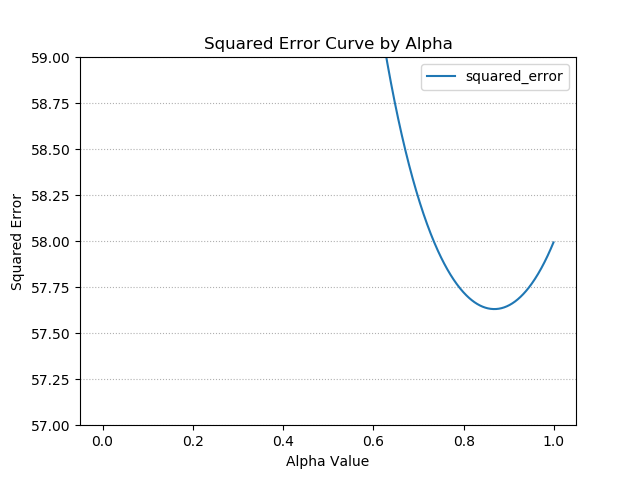We can see the minimum is right around 57.6. This matches with the work we did in Excel. We're almost there!

To find the minimum, first we have to define a formula that takes in a single variable. The variable needs to be the variable to be optimized - in this case, α.

```def totalSquaredError(alpha):
tot_sq_err = sum([pow(exponentialError(i, alpha),2) for i in range(1,len(df2['Period'])+1)])
```

Now we optimize using scipy.optimize.minimize_scalar:

```from scipy.optimize import minimize_scalar
glob_min = minimize_scalar(totalSquaredError, bounds=(0, 1), method='Golden')
print(glob_min)
```

And boom, we have our results!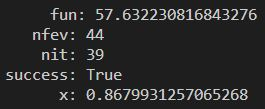That's it for the stationary time series models! Wow! We've already done a little bit of data science using that optimization algorithm.

Next Steps

In the next blog, I'll be looking at models for analyzing time series data with trends. I'll keep you posted!